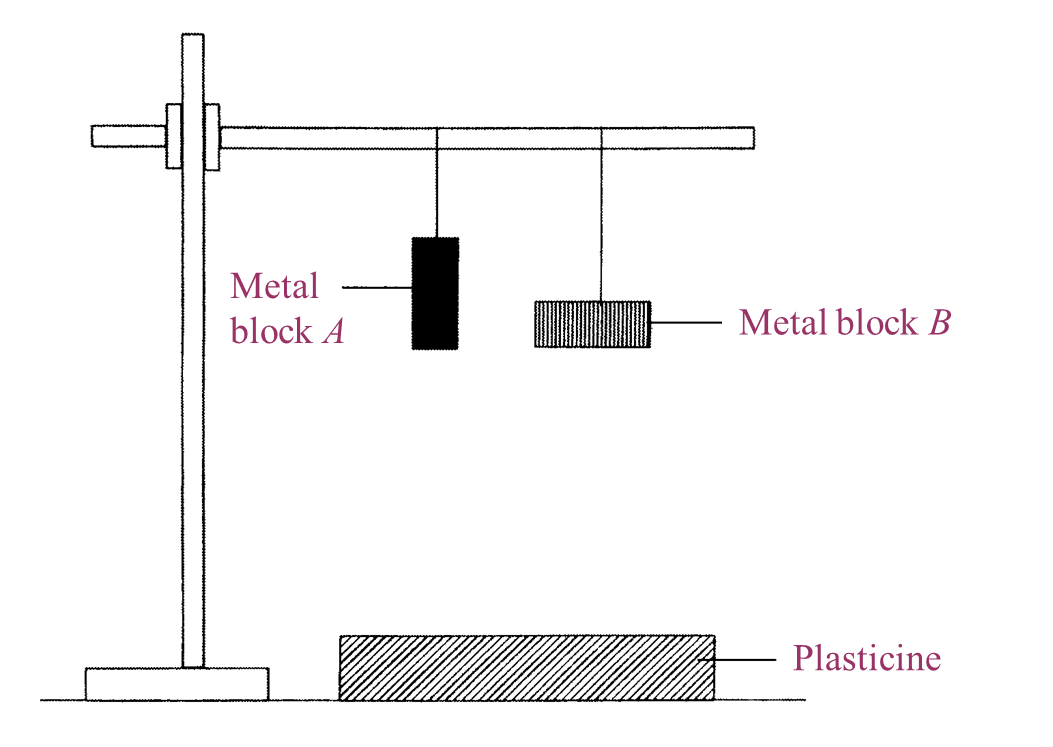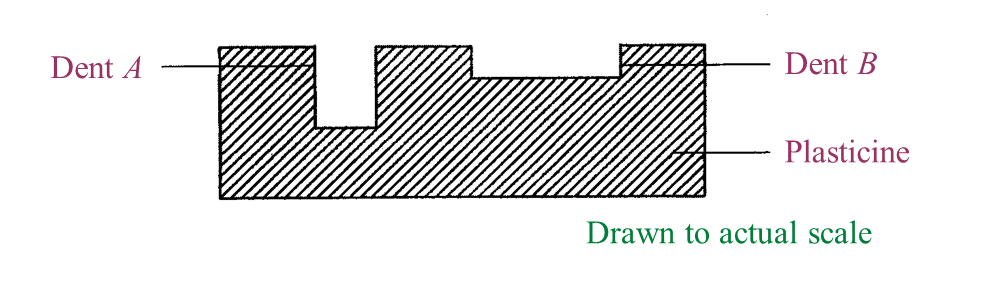# 5.7.2 The Concept of Pressure (Structured Questions)

Question 1:
Figure 1.1 shows an experiment to study the pressure produced by metal blocks, A and B of the same mass.Figure 1.1

Figure 1.2 shows the effect on the plasticine when metal blocks, A and B were dropped.Figure 1.2

(a)
What is the controlled variable in this experiment? [1 mark]

(b)
Write down one observation from the result of this experiment. [1 mark]

(c)
State one inference that can be made based on the observation in Figure 1.2. [1 mark]

(d)
Based on Figure 1.2, measure and record the depth of dent A. [1 mark]

(e)
Metal block C in Figure 1.3 has the same mass as metal blocks, A and B.Figure 1.3

Predict the depth of dent produced on the plasticine when metal block C is dropped from the same height. [1 mark]

(a)
The weight of the metal block (dropped from the same height).

(b)
The depth of dent A is more than the depth of dent B.

(c)
The surface area of A is smaller than the surface area of B.

(d)
The depth of dent A is 1 cm.

(e)
Deeper than dent A (or more than 1 cm).